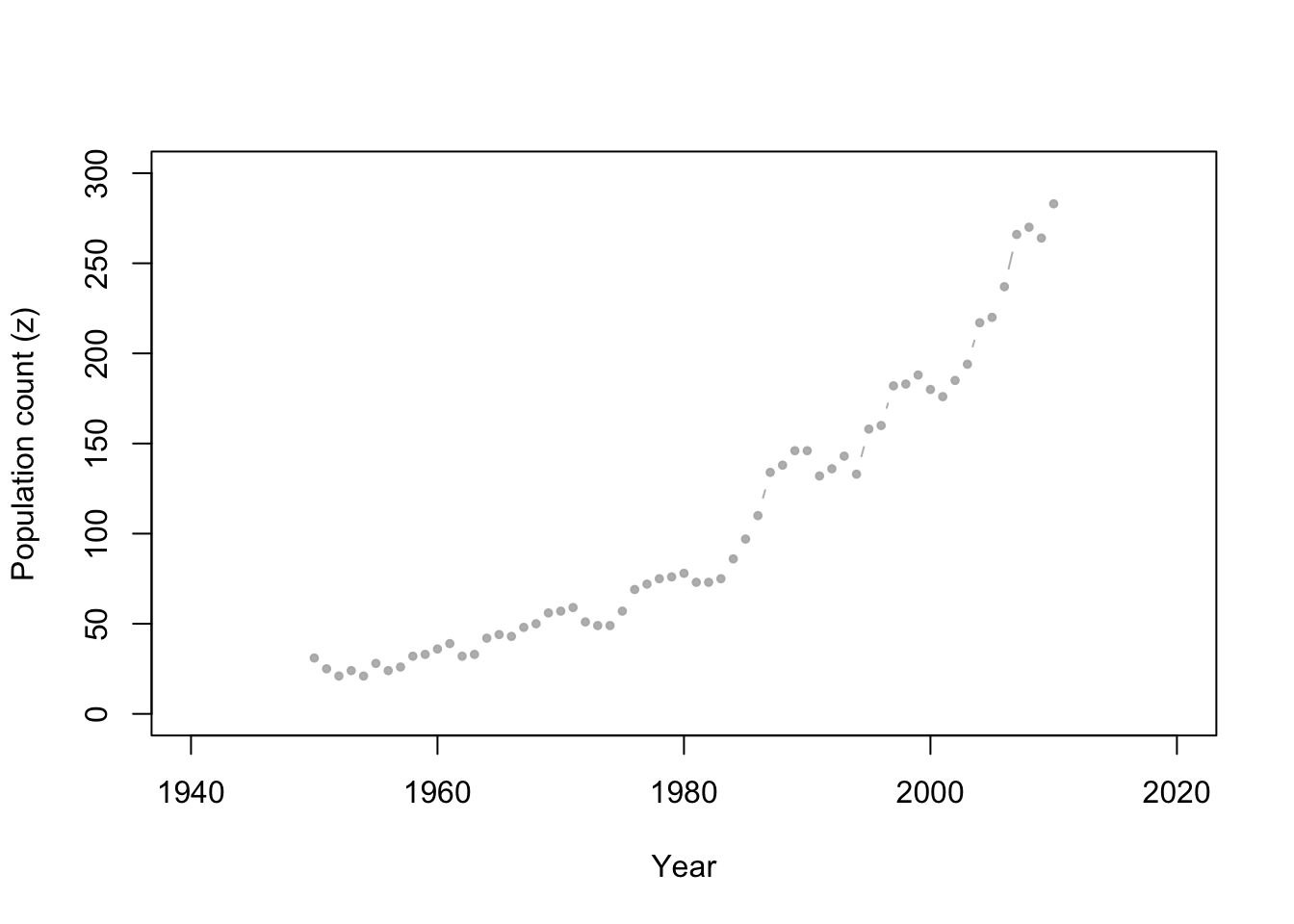# 4 August 27

## 4.1 Announcements

• Face-to-face office lectures and meetings KSU data
• Office hours 9:30 - 10:30 today or by appointment
• Assignment 2
• Self introductions
• List of potential collaborators is on Canvas (under files)

## 4.2 Bayesian hierarchical models

• During this course we will implement many models using the Bayesian hierarchical framework
• Today is a crash course on Bayesian statistics
• It is critical that you understand the concepts that we cover today
• Study technical note 1.1 on pg. 13 of Spatio-temporal statistics with R
• The Bayesian hierarchical modeling framework

$\text{Data model:} \;\;[\mathbf{z}|\mathbf{y},\boldsymbol{\theta}_{D}]$ $\text{Process model:} \;\;[\mathbf{y}|\boldsymbol{\theta}_{P}]$ $\text{Parameter model:} \;\;[\boldsymbol{\theta}]$

• Given a Bayesian hierarchical model we want the following:
• The posterior distribution of the parameters $$[\boldsymbol{\theta}|\mathbf{z}]$$
• The posterior predictive distribution $$[\mathbf{z}_{\text{pred}}|\mathbf{z}]$$
• Using Bayes’ theorem… $[\boldsymbol{\theta}|\mathbf{z}]=\int\frac{[\mathbf{z}|\mathbf{y},\boldsymbol{\theta}][\mathbf{y}|\boldsymbol{\theta}][\boldsymbol{\theta}]}{\int\int\mathbf{[z}|\mathbf{y},\boldsymbol{\theta}][\mathbf{y}|\boldsymbol{\theta}][\boldsymbol{\theta}]d\mathbf{y}d\mathbf{\boldsymbol{\theta}}}d\mathbf{y}$ $[\mathbf{z}_{\text{pred}}|\mathbf{z}]=\int\int\mathbf{[z}_{\text{pred}}|\mathbf{y},\boldsymbol{\theta}][\mathbf{y}|\boldsymbol{\theta}][\boldsymbol{\theta}|\mathbf{z}]d\mathbf{y}d\mathbf{\boldsymbol{\theta}}$

### 4.2.1 Motivating data example

• Whooping cranes
• Data set

url <- "https://www.dropbox.com/s/ihs3as87oaxvmhq/Butler%20et%20al.%20Table%201.csv?dl=1"

plot(df1$Winter, df1$N, xlab = "Year", ylab = "Population count (z)", xlim = c(1940,
2020), ylim = c(0, 300), typ = "b", cex = 0.8, pch = 20, col = rgb(0.7,
0.7, 0.7, 0.9))• We want to build a statistical model that enables
• Predictions and forecasts of the true population size
• Statistical inference on the date when the population will be larger than 1000 individuals
• Points to consider
• Whooping cranes are counted from an airplane (could some individuals be missed?)
• Aggregation of a spatio-temporal point pattern
• Are there any existing models that could work for these data?
• Anything else?

### 4.2.2 The data model

• The generic data model is $$[\mathbf{z}|\mathbf{y},\boldsymbol{\theta}_{D}]$$
• What is $$\mathbf{z}$$?
• What is the process $$\mathbf{y}$$?
• What is the support of $$\mathbf{z}$$ and $$\mathbf{y}$$?
• What distribution should we use for a data model?
• Let’s try $$[z_{t}|y_{t},p]\equiv\text{Binomial}(y_{t},p)$$
• Live demonstration in R
• What mathematical model should we use?
• How would the mathematical model control the moments of the PDF/PMF of the data model?

### 4.2.3 The process model

• The generic process model is $$[\mathbf{y}|\boldsymbol{\theta}_{P}]$$
• What distribution should we use for a process model?
• What is the support of $$\mathbf{y}$$?
• Let’s try $$[z_{t}|\lambda_{t}]\equiv\text{Poisson}(\lambda_{t})$$
• Live demonstration in R
• What mathematical model should we use?
• Study technical section 1.2 (pgs. 7-10) of Spatio-temporal statistics with R
• Descriptive mathematical model: $\lambda_{t} = e^{\beta_0+\beta_{1}t}$
• Dynamic mathematical model: The number of whooping cranes at any given time ($$t$$) can be constructed by $\begin{equation} \lambda(t+\Delta t)=\lambda(t)+b(t)-d(t) . \tag{3.1} \end{equation}$ At time $$t$$, let the births equal $$b(t)=\beta\Delta t\lambda(t)$$ and deaths equal $$d(t)=\alpha\Delta t\lambda(t)$$. Then write (3.2) as $\begin{equation} \lambda(t+\Delta t)=\lambda(t)+\beta\Delta t\lambda(t)-\alpha\Delta t\lambda(t). \tag{3.2} \end{equation}$ Now define the growth rate as $$\gamma=\beta-\alpha$$ and rewrite (3.2) as $\begin{equation} \lambda(t+\Delta t)=\lambda(t)+\gamma\Delta t\lambda(t). \tag{3.3} \end{equation}$ Next write (3.3) as $\begin{equation} \frac{\lambda(t+\Delta t)-\lambda(t)}{\Delta t}=\gamma\lambda(t) \tag{3.4} \end{equation}$ Take the limit of (3.4) as $$\Delta t\rightarrow0$$. $\begin{equation} \lim_{\Delta t\rightarrow0}\frac{\lambda(t+\Delta t)-\lambda(t)}{\Delta t}=\gamma\lambda(t) \tag{3.5} \end{equation}$ Finally replace $$\lim_{\Delta t\rightarrow0}\frac{\lambda(t+\Delta t)-\lambda(t)}{\Delta t}$$ in (3.5) with the differential operator to get $\begin{equation} \frac{d\lambda(t)}{dt}=\gamma\lambda(t). \tag{3.6} \end{equation}$ The analytical solution to (3.6) is $\begin{equation} \lambda(t)=\lambda_{0}e^{\gamma (t-t_0)}\ \tag{3.7} \end{equation}$

### 4.2.4 The parameter model

• The final step is to specify PDFs/PMFs for the parameters
• In what follows we will use the Binomial data model and Poisson process model (with the exponential growth mathematical model)
• What parameter models should we use?

### 4.2.5 Simulating data from the prior predictive distribution

• The prior predictive distribution is capable of providing predictions/forecasts without the use of any data
• Other fields call this “simulation modeling” or a “sensitivity analysis”
• It is basically data free statistics (i.e., prediction, forecasts, and inference is 100% assumption driven)
• Used as a form of model (assumption) checking in Bayesian statistics
• Super easy to do and helps us “prototype” our statistical model before we put in any more work
• Live demonstration in R

### 4.2.6 Model fitting

• Class discussion
• We will cover this in future lectures